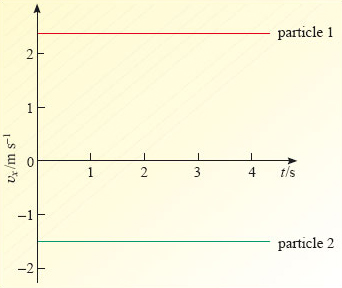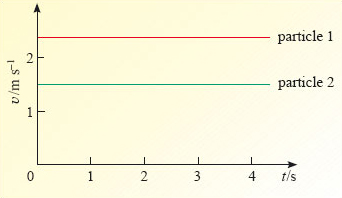Describing motion along a line

This free course is available to start right now. Review the full course description and key learning outcomes and create an account and enrol if you want a free statement of participation.

Free course

# 3.5 Velocity-time and speed-time graphs

Just as we may plot the position-time graph or the displacement-time graph of a particular motion, so we may plot a velocity-time graph for that motion. By convention, velocity is plotted on the vertical axis (since velocity is the dependent variable) and time (the independent variable) is plotted on the horizontal axis. In the special case of uniform motion, the velocity-time graph takes a particularly simple form - it is just a horizontal line, i.e. the gradient is zero. Examples are given in Figure 17; notice that the velocity can be positive or negative, depending on the direction of motion.Figure 17: Velocity-time graphs for uniform motion

Rather than plotting the velocity it is sometimes useful to plot the magnitude of the velocity, in other words, the speed. The resulting plots are speed-time graphs and examples are shown in Figure 18. Notice how all of the speeds are positive; in particular, the velocity of −1.5 m s−1 in Figure 17 corresponds to a speed of 1.5 m s−1 in Figure 18.

Clearly, a speed-time graph provides less information than a velocity-time graph, but it may be sufficient. The next time you buy a Ferrari, you may well enquire about its top speed, but you are unlikely to ask 'in which direction?'Figure 18: Speed-time graphs for uniform motion, corresponding to the velocity-time graphs shown in Figure 17
S207_2